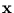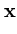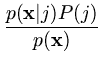Next: A.2 Maximum likelihood Up: A. Statistical tools Previous: A. Statistical tools

# A.1 Bayes' theorem

The probability p(, j) of observing bothand j can be written in two ways,

 p(, j) = p(| j)P(j) = P(j|)p() . (A.1)

p() is the probability of(independent of j), P(j) is the probability of j (independent of), p(| j) is the probability ofunder the condition that j is given, and P(j|) is the probability of j under the condition thatis given. Reorganizing (A.1) about P(j|) gives

 P(j|) =. (A.2)

This is Bayes' theorem.

Heiko Hoffmann
2005-03-22